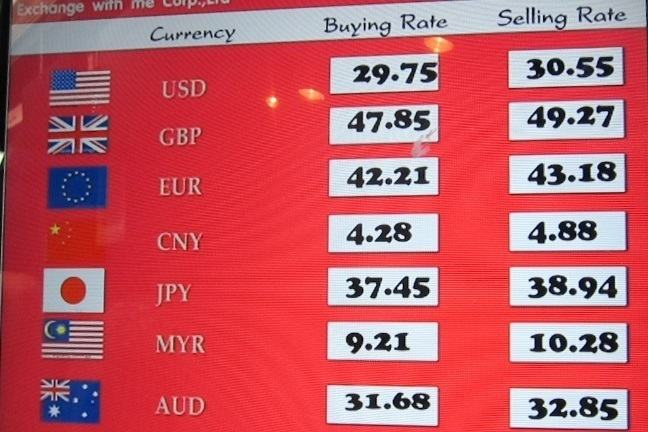1.22

## UNSW SydneyYour pounds or my dollars?

# Conversion between different units

Whenever we have two or more systems of measuring, conversion questions come up. These give us a rich natural family of examples for direct proportionalities. But in fact there are two fundamentally different kinds of conversions: between different units in the same system, and between different systems.

In this lesson, you will

• get an overview of some useful conversions

• learn how to mathematically think about the problem of converting from one unit to another.

## Different units within the same system

Within a given system, there are often many different kinds of units, designed for convenience at different scales. Also there is often quite a high degree of historical whimsiness involved.

The British Imperial system of lengths in based on the foot, which traces back to the ancient Egyptians, and was passed on to the Greeks and Romans. The introduction of the yard as a unit of length came later. Some believe the origin was the double cubit, from the Egyptian, but was in any case standardized as the distance from the tip of the nose to the end of the thumb of King Henry I (reigned 1100–1135). Here are some common equivalences:

Imperial Length Proportionalities
12 inches = 1 foot
3 feet = 1 yard
1760 yards = 1 mile

There are also links, chains, furlong and poles but these are more specialized and not in common usage these days.

In the metrical system, everything is based on powers of ten, with

Metric Length Proportionalities
10 millimetres = 1 centimetre
100 centimetres = 1 metre
1000 metres = 1 kilometre

If we want to convert say $\normalsize{100}$ yards to inches, what would we do? We employ basic proportionalities: $1\,\mbox{ yard} = 3\,\mbox{ feet}$ and $1 \,\mbox{ foot} = 12 \,\mbox{ inches}$. These equalities can be combined as:

So $1 \, \mbox{ yard} = 36\,\mbox{ inches}$. Multiplying this by $100$ gives the desired conversion:

Q1 (E): Convert $\normalsize{200}$ yards to miles.

Q2 (E): Convert $\normalsize{300}$ kilometres to centimetres.

Another way of performing this conversion is to use direct proportionality. If we let $x = \mbox{yards}$ and $y = \mbox{inches}$, then a change of one yard corresponds to a change of 36 inches and

and the equation of this line is $y=36 x$. When $x=100$, then $y = 3600$.

## Converting from one system to another

If we want to convert from British Imperial to metrical units, we need to know how to convert. These are usually decided by international agreement and are not as neat as the conversions between units in the same system.

International Agreement Date of Agreement
1 inch = 2.54 centimetres 1896
1 mile = 1.609344 kilometres 1959

So if we want to convert $\normalsize{30}$ inches to metres, we could proceed as follows: let $y=\mbox{centimetres}$ and $x=\mbox{inches}$. A change in one inch corresponds to a change in 2.54 centimetres:

So our direct proportionality between centimetres and inches is $y = 2.54\, x$. If we let $z = \mbox{ metres}$ the direct proportionality between metres ($z$) and centimetres ($y$) can be expressed as:

Combining these equations gives:

Q3 (E): Substitute $x=30$ into the above equation to convert 30 inches to metres.

Q4 (M): Convert $\normalsize{14,000}$ feet to kilometers.

Q5 (C): Convert $\normalsize{46}$ miles/hour to metres/sec.